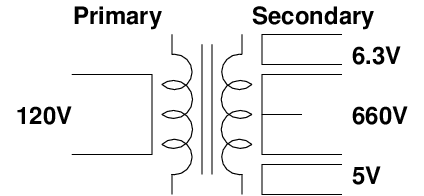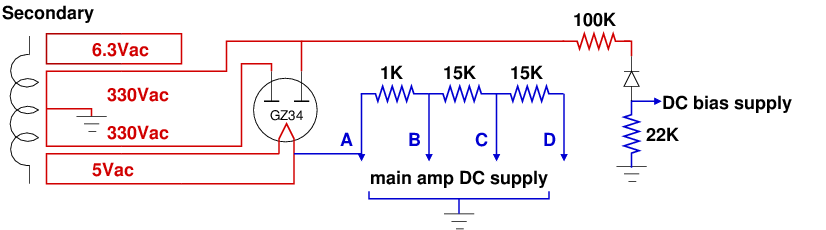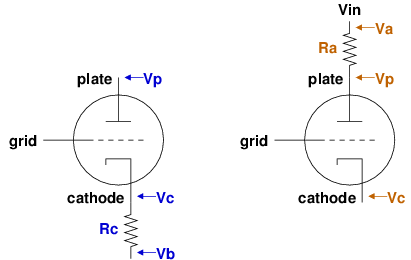# Mojotone Princeton Reverb Clone Project

Here are some measurements from the tests I did as I checked the amplifier.

## Power supply with no load120 Vac power is supplied to the amplifier through the mains power cord. The mains current is routed through the fuse and power switch into the primary winding of the power transformer. Current in the primary winding of the transformer generates AC voltage on the secondary side of the transformer. The secondary side of the power transformer has three taps: a 5 Vac supply for the rectifier, a 6.3 Vac supply for heating the filaments of the preamp and power tubes, and a 660 Vac high voltage supply for the rest of the amp. The 660 Vac windings on the secondary side of the power transformer have a center tap that we can connect to ground to provide us with two 330 Vac supplies.

The next two figures show the 5 Vac and 6.3 Vac, respectively. With no load on the power supply the 5 Vac supply measures 5.45 Vac and the 6.3 Vac supply measures 6.82 Vac.

The next figure shows the two legs of the 660 Vac supply with the ground on the center tap. The sine waves clip because the voltage higher than the max voltage that can be measured by the scope's 10x probes. 100x probes are required to measure this voltage.

The next two figures show the high voltage power supply using 100x probes. The first figure shows the voltage between the two ends of the power supply (note that the scale is 500V per block). With no load we see 675 Vac. The next figure (at scale 200V per block) shows the two legs of the high voltage supply with the ground at the center tap. The legs roughly measure 345 Vac.

The next two plots shows the voltages at the ends of high-voltage windings and the 6.3 Vac supply winding with respect to ground.

## Power supply voltagesThe secondary side of the power transformer provides has three AC taps. The AC circuits are shown in red in the figure above. The 6.3Vac circuit provides power for the pilot light and the heaters for all the tubes except the rectifier tube. Both legs of the 6.3Vac circuit are wired through a 100 ohm resistor to the chassis ground (not shown in the figure). This connection is to provide a voltage reference to chassis ground and ensure that the two legs are properly out of phase to cancel out hum (the AC current does not flow to ground).

The 5Vac circuit is for heating the filament of the GZ34 rectifier tube. The AC current flows from one side of the 5Vac tap on the power transformer through the GZ34 and back to the other side of the 5Vac tap.

The high voltage 660Vac windings on the secondary side of the power transformer have a center tap connected to the chassis ground. This results in the 660Vac voltage being divide into two 330Vac supplies with respect to the chassis ground. The two 330Vac supplies are 180 degrees out of phase with each other. This can be seen in the oscilloscope plots above.

### Rectification

Rectification is converting AC current (e.g. the sine wave) into DC current (flat, like what you would get from a battery). The Princeton rectifies AC current (shown above in red) to DC current (show in blue) in two places. First, the two plates inside the GZ34 rectifier tube only allow current in the high-voltage circuit to flow in one direction. Since both 330Vac legs are attached to the GZ34's plates and the two legs are 180 degrees out of phase, we end up with only the top halves of each sine wave passing through the GZ34. Thus, the output of the GZ34 is DC current (but choppy, since the sine waves are not flat). Capacitors are used to smooth out the bumps in this choppy DC current. The DC current produced by the rectifier tube flows through the same wire used to provide the 5Vac heater supply for the tube, but instead of flowing back into the power transformer it flows through the main part of the amplifier to reach its ground (the chassis ground). The voltage of the DC output of the rectifier is high. This DC voltage is often called the "B+" voltage. We reduce the high DC voltage in steps by passing it through a series of resistors. Each leg of the DC supply is identified by a letter: the highest voltage is at node "A" and the lowest DC power supply voltage is at node "D." Additional capacitors (not shown) are used to further smooth the waveform as we reduce the voltage. The capacitors used in this part of the amplifier are the ones that can hold a significant charge even after the amp is switched off and should be drained to ground before working on the internals of the amplifier.

The second place we rectify AC current into DC current in the Princeton Amp is in the DC bias power supply circuit. One leg of the 330Vac supply from the transformer is connected to a single diode (after being passed through a 100K resistor to reduce the voltage). This diode is set to only allow the negative part of the AC supply through. This is called a "half-wave rectifier" circuit. The DC output of the diode is connected to a capacitor (not show, but its purpose is to smooth the ripples in the DC output) and a 22K resistor to produce a negative DC voltage used to bias the 6V6 power tubes (more on that later).

### Voltage Measurements

Here are some voltage measurements from the amp after the build was completed. First up is measurements of the AC voltage from the secondary side of the power transformer (the primary was plugged in to an isolation transformer generating 119.4 Vac). I measured it with no tubes installed, with just the rectifier tube installed, and will all the tubes installed. The voltages drop slightly as load is added to the amp.

circuitNo tubes installed (Vac) Rectifier only (Vac) All tubes installed (Vac)
rectifier heater (pin 2 to 8) 5.365.205.02
heaters (pins 4 and 5 to 9)6.766.766.26
high voltage (GZ34 pin 4 to ground) 335335321
high voltage (GZ34 pin 6 to ground) 335335321

Next we have the the DC power at nodes A, B, C, and D. Without the rectifier tube installed there is no current load on the power supply so the voltage is almost constant (no current means no voltage drop over the resistors). All voltages are measured with respect to ground unless noted otherwise.

NodeRectifier only (Vdc)All tubes installed (Vdc)
A466392
B466381
C465310
D465257

The DC bias supply receives its voltage from the AC high voltage section of the power transformer. The current flows through the 100K resistor, then through the half-wave rectifier diode, and on to a 22K resistor. Since only AC voltage is present at the input to the 100K resistor, the DC voltage there is close to zero. The voltage on diode side of the 22K resistor is used to bias the power tubes (the other side of the 22K resistor is tied to ground). With no tubes installed in the amp the voltages before and after the 100K resistor are 335 Vac and 199 Vac respectively. The DC voltages are in the following table.

circuit locationNo tubes installed (Vdc) Rectifier only (Vdc) All tubes installed (Vdc)
input to 100K resistor -0.016-0.016-0.017
input to diode129.4129.7122
output of diode -38.0-37.9-36.7
6V6 grids (pin 5) -32.3-32.1-31

## Tube power dissipation and bias voltages measurements

Properly operating vacuum tubes have a constant DC current flowing through them. The AC audio signal is then superimposed on this DC current. The amount of DC current flowing through a tube is controlled by the bias voltage. If the bias current is too low, the tube is said to be running "cold" and is not dissipating much power. On the other hand, if the bias current is too high, the tube is running "hot" and may be dissipating too much power. Tubes that are biased too cold may not produce good sounding audio, while tubes that are biased too hot may have reduced lifetime. A tube's data sheet should indicate the recommended power dissipation for that type of tube (in units of watts).

The figure below shows the two main types of components inside the tubes used by the Princeton amp. The 12AX7 and 12AT7 tubes contain two triodes in a tube, while the 6V6 power tubes contain one pentode. (The connections for filaments heaters are not shown.) The DC bias current flows from the plate through the tube to the cathode as electrons are emitted from the cathode to the plate. The bias current is controlled by the DC voltage between the cathode and grid. Under normal operation the grid's voltage with respect to ground should less than the cathode's voltage with respect to ground. The more negative the DC voltage at the grid is to the DC voltage at the cathode, the less DC bias current will flow through the tube. The less current that flows, the lower the tube's power dissipation will be. If the grid's DC bias voltage gets too close the cathode's voltage, too much current will flow through the tube and it may get too hot ("red plate") and burn out quickly.Tubes in the Princeton amp are biased in one of two ways. The 12AX7s and 12AT7 are cathode biased. At a high-level this means that the cathode is connected to a resistor and the other end of that resistor is connected to a point in common with the grid (often ground). This causes the voltage at the cathode to be higher than the voltage at the grid thus giving us our bias voltage. The larger the resistor is, the greater the bias voltage is and the less current will flow through the tube. Note that there may also be a capacitor connected to the cathode, but since capacitors block DC current they will have no impact on the bias voltages.

The other way tubes are biased in the Princeton amp is fixed bias The two 6V6 power tubes use this type of bias. In the Princeton fixed bias circuit the cathodes of the power tubes are connected directly to ground and an independent power supply is used to apply a negative voltage to the grid. (Since the cathode is tied to ground at zero volts, the grid voltage must be less than this to control the tube's power dissipation.) The DC bias voltage is supplied by the diode in the half-wave rectifier discussed earlier. The 22K resistor controls the value of the DC bias voltage. If this resistor is made larger, the DC bias voltage will become more negative with respect to the cathode and the DC bias current will be reduced. Reducing the value of the 22K resistor will have the opposite effect (i.e. it will make the tube run hotter). In the Fender Princeton amp design, the only way to change the bias voltage on the 6V6 tubes is to replace the 22K resistor with a resistor with a different value. To make the bias easier to adjust, you can replace the 22K resistor with a circuit that contains a potentiometer that changes the resistance (I made this change to my amp).

### Measuring power dissipation

To measure power dissipation we use:
• Ohm's Law: Voltage = Current * Resistance (V=IR)
• the Power Law: Power = Voltage * Current (P=VI)
Where Voltage is measured in Volts, Current in Amps, Resistance in Ohms, and Power in Watts. There are two steps: first we compute the DC bias current flowing through the tube using Ohm's law, then we use that current along with the voltage drop across the tube in the Power law to compute the power dissipation in watts. The following figure shows two options for this process:In all cases we use three voltage measurements and one resistance value to compute the power dissipation. For cathode biased tubes it is convenient to use the cathode resistor Rc value to compute the DC current (note that any capacitor in parallel with resistor can be ignored because it should not be carrying any DC current). Thus we use the blue values on the left side of the figure to compute the power dissipation like this:

• tube current: I=V/R => (Vc - Vb) / Rc
• tube power (using I from above): P=VI => (Vp - Vc) * I
For fixed biased tubes that have their cathode tied to ground we need a difference place to measure the resistance to compute the current. The 6V6 power tube's plates are directly connected to the output transformer primary coil. We can use the resistance from the center tap of the output transformer primary to the plate as Ra to compute the current, as shown in brown on the right side of the figure. This results in:
• tube current: I=V/R => (Va - Vp) / Ra
• tube power (using I from above): P=VI => (Vp - Vc) * I
With this in mind, we can now measure the power dissipation of all the tubes.

### Power dissipation measurements

The power specifications for the Princeton's tubes are:
• 7025/12AX7: 1.2 watts max
• 12AT7: 2.8 watts max
• JJ 6V6: 14 watts max

For the 9 pin tubes, from the rear from left to right we have 12AX7(1), 12AX7(2), 12AT7, and 7025 (which is also a 12AX7). All these tubes are cathode biased. Each tube has two triodes it in, so we need to check the dissipation for both sides, except for the 12AT7 which has both sides wired together sharing the same cathode resistor. So for the 12AT7 we have to divide the computed current in half (assuming that it is equally split between each triode). The results for the first side are:

Item12AX7(1)12AX(2)12AT77025
Triode (T1) purposevibrato lfo reverb recoveryreverbinput preamp
T1 plate (pin 1, Vdc) 227167337164.7
T1 grid (pin 2, Vdc) 0000
T1 cathode (pin 3, Vdc) 2.271.367.351.347
T1 cathode resistor (ohms) 3300150022001500
T1 cathode resistor voltage (far end, Vdc) 0000
T1 current (amps) 0.0006880.0009070.0016700.000898
T1 power dissipation (watts) 0.1550.1500.6170.147

The results for the second side (except for the 12AT7 which is covered above) are:
Item12AX7(1)12AX(2)7025
Triode (T2) purposeinverter mixerafter volume pot
T2 plate (pin 6, Vdc) 236165.4171.7
T2 grid (pin 7, Vdc) 17.900
T2 cathode (pin 8, Vdc) 70.61.4481.302
T2 cathode resistor (ohms) 100015001500
T2 cathode resistor voltage (far end, Vdc) 69.70.04470
T2 current (amps) 0.0009000.0009360.000868
T2 power dissipation (watts) 0.1490.1530.148

All the 9 pin tube power dissipations are in the correct range for their tube types.

For the 6V6 power tubes we first measure the resistance of the output transformer from the center tap to each side of the winding (where it connects to the plate of one of the 6V6s). The output transformer uses a brown and a blue wire for each side of the winding, so we use those colors to identify which 6V6 tube we are measuring. The results are:

Item6V6 (brown wire)6V6 (blue wire)
center tap to plate voltage drop (Vdc) 3.704.15
plate (pin 3, Vdc) 386386
screen (pin 4, Vdc) 381381
grid (pin 5, Vdc) -31.3-31.3
cathode (pin 8, Vdc) 00
center tap to plate resistance (ohms) 142.1159.1
current (amps) 0.0260380.0260842
power dissipation (watts) 10.05110.069

The maximum power dissipation for the 6V6 tubes is 14W, but for tubes in this configuration it is recommended that you run them at about 70% of the max wattage. 70% of 14W is 9.8W, so our tubes are running slightly hotter than this at 10W. This is fine.